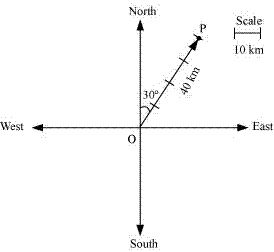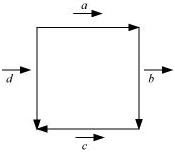# Class 12 Maths NCERT Solutions for Chapter 10 Vector Algebra Exercise 10.1### Vector Algebra Exercise 10.1 Solutions

1. Represent graphically a displacement of 40 km, 30° east of north.

SolutionHere, vector OP represents the displacement of 40 km, 30° East of North.

2. Classify the following measures as scalars and vectors.
(i) 10 kg
(ii) 2 metres north-west
(iii) 40°
(iv) 40 watt
(v) 10–19 coulomb
(vi) 20 m/s2
Solution

(i) 10 kg is a scalar quantity because it involves only magnitude.
(ii) 2 meters north-west is a vector quantity as it involves both magnitude and direction.
(iii) 40° is a scalar quantity as it involves only magnitude.
(iv) 40 watts is a scalar quantity as it involves only magnitude.
(v) 10–19 coulomb is a scalar quantity as it involves only magnitude.
(vi) 20 m/s2 is a vector quantity as it involves magnitude as well as direction.

3. Classify the following as scalar and vector quantities.
(i) time period
(ii) distance
(iii) force
(iv) velocity
(v) work done

Solution

(i) Time period is a scalar quantity as it involves only magnitude.
(ii) Distance is a scalar quantity as it involves only magnitude.
(iii) Force is a vector quantity as it involves both magnitude and direction.
(iv) Velocity is a vector quantity as it involves both magnitude as well as direction.
(v) Work done is a scalar quantity as it involves only magnitude.

4. In Figure, identify the following vectors.(i) Coinitial
(ii) Equal
(iii) Collinear but not equal

Solution

(i) Vectors a⃗ and  d⃗ are coinitial because they have the same initial point.
(ii) Vectors b and d are equal because they have the same magnitude and direction.
(iii) Vectors a and c⃗ are collinear but not equal. This is because although they are parallel, their directions are not the same.

5. Answer the following as true or false.
(i) a⃗ and -a⃗ are collinear.
(ii) Two collinear vectors are always equal in magnitude.
(iii) Two vectors having same magnitude are collinear.
(iv) Two collinear vectors having the same magnitude are equal.
Solution

(i) True.
Vectors  a⃗ and -a⃗  are parallel to the same line.
(ii) False.
Collinear vectors are those vectors that are parallel to the same line.
(iii) False.
It is not necessary for two vectors having the same magnitude to be parallel to the same line.
(iv) False.
Two vectors are said to be equal if they have the same magnitude and direction, regardless of the positions of their initial points.